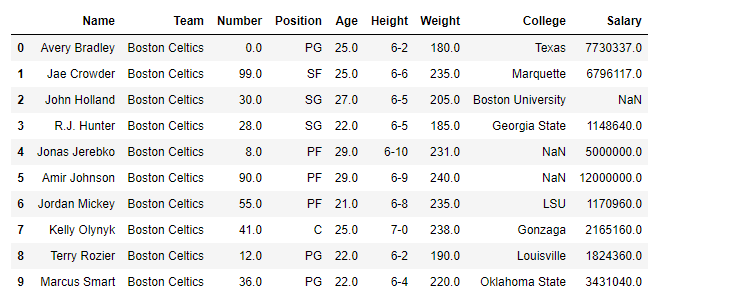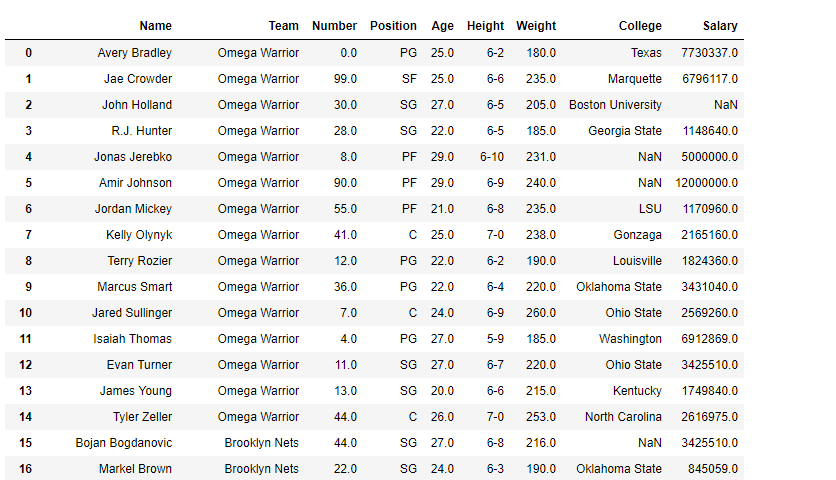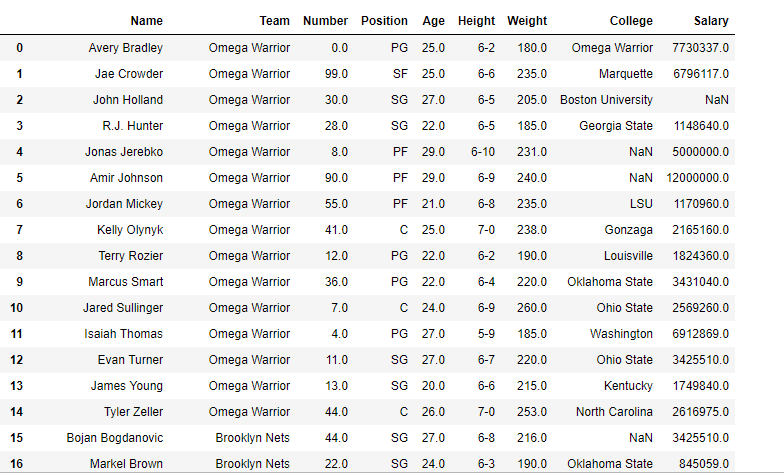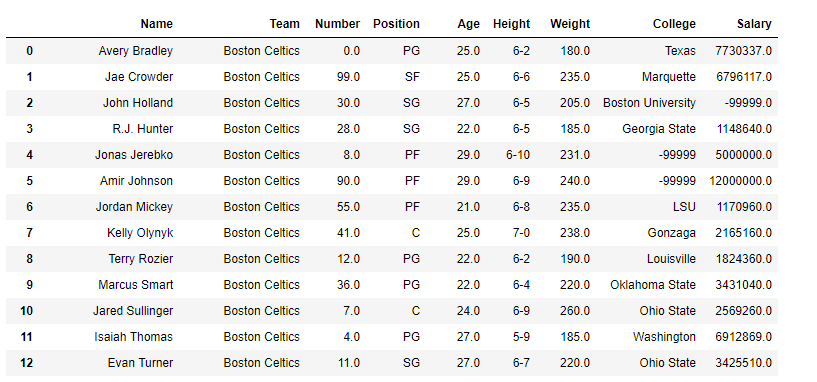# Python | Pandas dataframe.replace()

Pandas dataframe.replace() function is used to replace a string, regex, list, dictionary, series, number, etc. from a Pandas Dataframe in Python. Every instance of the provided value is replaced after a thorough search of the full DataFrame.

### Syntax of dataframe.replace()

Syntax: DataFrame.replace(to_replace=None, value=None, inplace=False, limit=None, regex=False, method=’pad’, axis=None)

Parameters:

• to_replace : [str, regex, list, dict, Series, numeric, or None] pattern that we are trying to replace in dataframe.
• value : Value to use to fill holes (e.g. 0), alternately a dict of values specifying which value to use for each column (columns not in the dict will not be filled). Regular expressions, strings and lists or dicts of such objects are also allowed.
• inplace : If True, in place. Note: this will modify any other views on this object (e.g. a column from a DataFrame). Returns the caller if this is True.
• limit : Maximum size gap to forward or backward fill
• regex : Whether to interpret to_replace and/or value as regular expressions. If this is True then to_replace must be a string. Otherwise, to_replace must be None because this parameter will be interpreted as a regular expression or a list, dict, or array of regular expressions.
• method : Method to use when for replacement, when to_replace is a list.

Returns: filled : NDFrame

### Example:

Here, we are replacing 49.50 with 60.

## Python3

 `import` `pandas as pd ` ` `  `df ``=` `{ ` `  ``"Array_1"``: [``49.50``, ``70``], ` `  ``"Array_2"``: [``65.1``, ``49.50``] ` `} ` ` `  `data ``=` `pd.DataFrame(df) ` ` `  `print``(data.replace(``49.50``, ``60``)) `

Output:

```   Array_1  Array_2
0     60.0     65.1
1     70.0     60.0```

### Usages of Pandas dataframe.replace()

Here, we are going to see the implementation of dataframe.replace() methods.

## Python3

 `# importing pandas as pd ` `import` `pandas as pd ` ` `  `# Making data frame from the csv file ` `df ``=` `pd.read_csv(``"nba.csv"``) ` ` `  `# Printing the first 10 rows of the data frame for visualization ` `df[:``10``] `

Output:### Example 1:

We are going to replace team “Boston Celtics” with “Omega Warrior” in the ‘df’ Dataframe.

## Python3

 `# this will replace "Boston Celtics" with "Omega Warrior" ` `df.replace(to_replace``=``"Boston Celtics"``, ` `           ``value``=``"Omega Warrior"``) `

Output:### Example 2:

Replacing more than one value at a time. Using python list as an argument We are going to replace team “Boston Celtics” and “Texas” with “Omega Warrior” in the ‘df’ Dataframe.

## Python3

 `# importing pandas as pd ` `import` `pandas as pd ` ` `  `# Making data frame from the csv file ` `df ``=` `pd.read_csv(``"nba.csv"``) ` ` `  `# this will replace "Boston Celtics" and "Texas" with "Omega Warrior" ` `df.replace(to_replace``=``[``"Boston Celtics"``, ``"Texas"``], ` `           ``value``=``"Omega Warrior"``) `

Output:

Notice the College column in the first row, “Texas” has been replaced with “Omega Warriors”### Example 3:

Replace the Nan value in the data frame with the -99999 value.

## Python3

 `# importing pandas as pd ` `import` `pandas as pd ` ` `  `# Making data frame from the csv file ` `df ``=` `pd.read_csv(``"nba.csv"``) ` ` `  `# will replace Nan value in dataframe with value -99999 ` `df.replace(to_replace ``=` `np.nan, value ``=``-``99999``) `

Output:Notice all the Nan value in the data frame has been replaced by -99999. Though for practical purposes we should be careful with what value we are replacing nan value.

Whether you're preparing for your first job interview or aiming to upskill in this ever-evolving tech landscape, GeeksforGeeks Courses are your key to success. We provide top-quality content at affordable prices, all geared towards accelerating your growth in a time-bound manner. Join the millions we've already empowered, and we're here to do the same for you. Don't miss out - check it out now!